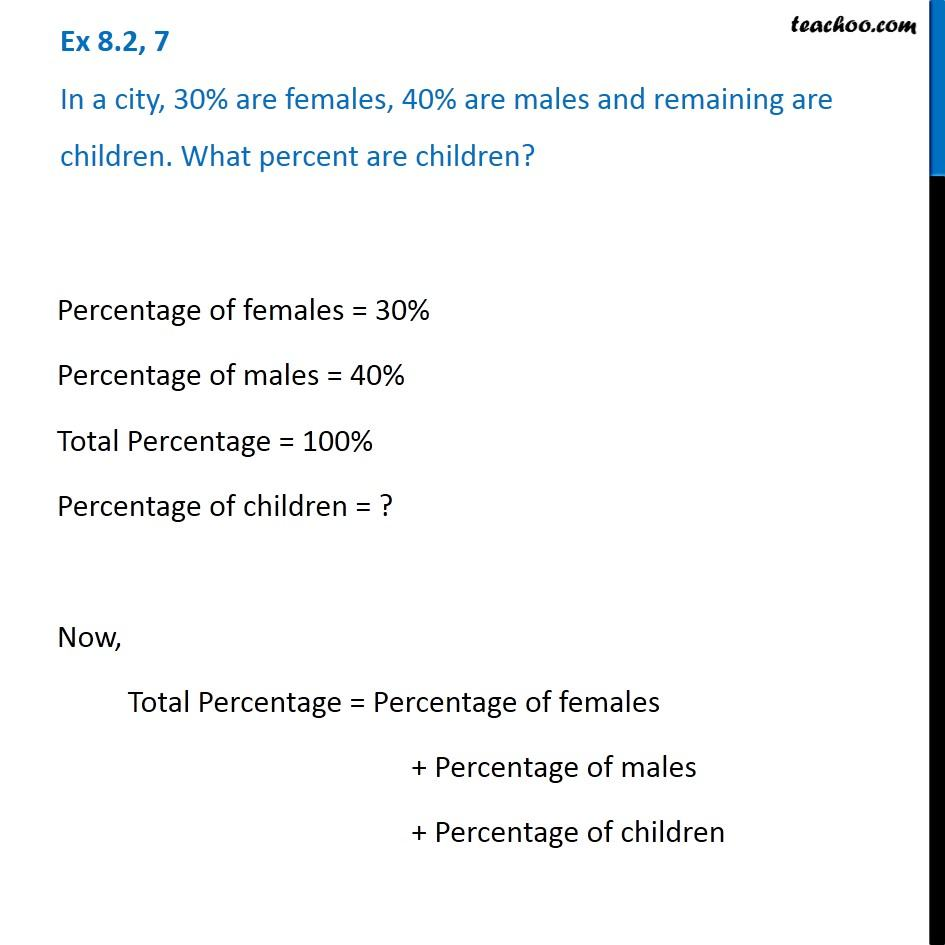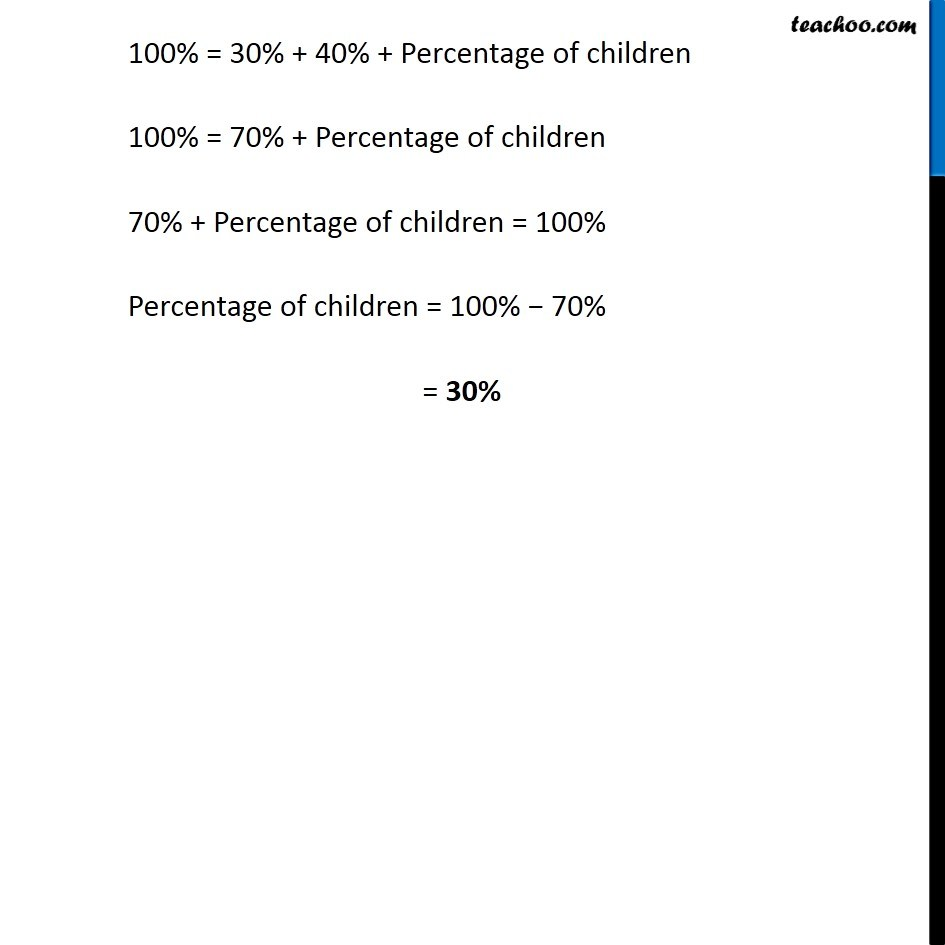Subscribe to our Youtube Channel - https://you.tube/teachoo

1. Chapter 8 Class 7 Comparing Quantities
2. Concept wise
3. Using percentage

Transcript

Ex 8.2, 7 In a city, 30% are females, 40% are males and remaining are children. What percent are children? Percentage of females = 30% Percentage of males = 40% Total Percentage = 100% Percentage of children = ? Now, Total Percentage = Percentage of females + Percentage of males + Percentage of children 100% = 30% + 40% + Percentage of children 100% = 70% + Percentage of children 70% + Percentage of children = 100% Percentage of children = 100% − 70% = 30%

Using percentage